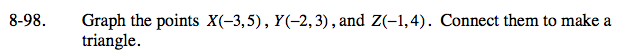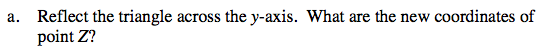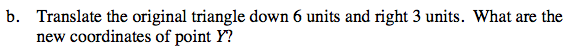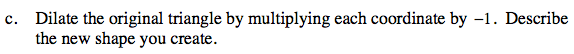Home > ACC7 > Chapter cc38 > Lesson cc38.2.3 > Problem8-98

8-98.
1. Graph the points X(−3, 5), Y(−2, 3), and Z(−1, 4). Connect them to make a triangle. 8-98 HW eTool (Desmos). Homework Help ✎

1. Reflect the triangle across the y‑axis. What are the new coordinates of point Z?

2. Translate the original triangle down 6 units and right 3 units. What are the new coordinates of point Y?

3. Dilate the original triangle by multiplying each coordinate by −1. Describe the new shape you create.Use the eTool below to graph the points and complete the remaining parts.
Click the link at right for the full version of the eTool: CC3 8-98 HW eTool